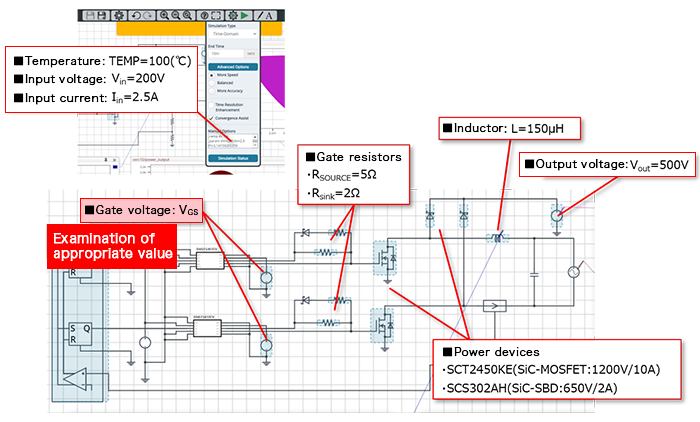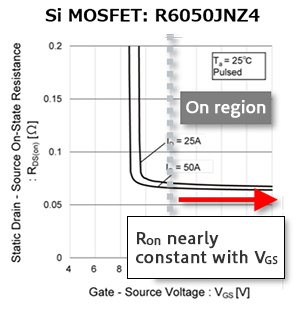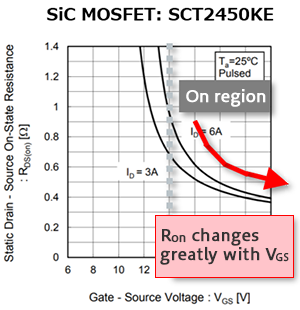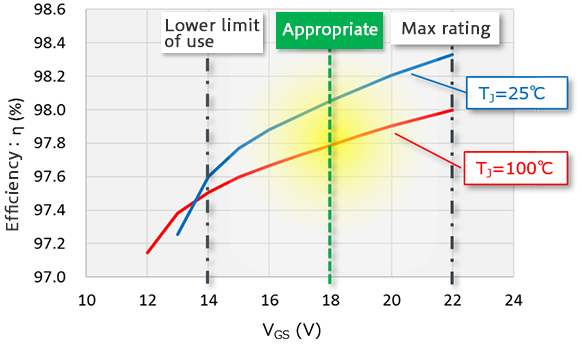# PFC Circuits: Examination of Appropriate Gate Driving Voltages

2021.08.18

・When a SiC MOSFET is used as a switching element, because the change in Ron with changes in the gate driving voltage VGS is considerable, the VGS setting is important.

・When studying the gate driving voltage VGS of a SiC MOSFET, a balance between efficiency and safety should be considered.

## PFC Circuits: Examination of Appropriate Gate Driving Voltages

Here, appropriate values for the gate driving voltage VGS of a SiC MOSFET that is to be used as a switching element in a PFC circuit are examined.

### Example Circuit

As an example circuit, the simulation circuit “A-2. PFC BCM Diode-Bridge-Less Vin＝200V Iin＝2.5A” in the Power Device Solution Circuit/AC-DC PFC list is used (see Fig. 9). Details of the circuit diagram can also be seen here.

In this example, simulations are used to examine an appropriate VGS value to drive the SCT2450KE SiC MOSFET shown in Fig. 9, which is the low-side switching element.Fig.9 : “A-2. PFC BCM Diode-Bridge-Less Vin＝200V Iin＝2.5A” PFC simulation circuit

### Relation between On Resistance and Gate Driving Voltage

As shown in Fig. 10, the on-resistance Ron of a conventional Si (silicon) MOSFET is essentially constant with respect to VGS while in the on state. In contrast, in the case of a SiC MOSFET Ron changes considerably depending on VGS, as shown in Fig. 11, and so the VGS setting is even more important than for a Si MOSFET. In other words, if the VGS value of a SiC MOSFET is too low, conduction losses increase and efficiency is worsened. Conversely, if VGS is set too high with the intention of improving efficiency, there is the concern that ratings may be exceeded. Hence it is extremely important that VGS be set appropriately.Fig. 10: Relation between Ron and VGS for a Si MOSFETFig. 11: Relation between Ron and VGS for a SiC MOSFET

### Examination of Appropriate Gate Driving Voltages

Fig. 12 shows the results of simulation of the efficiency when the value of VGS for the SiC MOSFET in the PFC circuit of Fig. 9 is changed.Fig. 12: Efficiency simulation results when the VGS of a SiC MOSFET is changed

When VGS is 14 V or lower, an increase in Ron causes the efficiency to drop sharply. This phenomenon is more prominent at lower temperatures; in this region there is a strong possibility of device failure, making use impossible. On the other hand, the higher the value of VGS, the better is the efficiency, but if the rated value (VGS = 22 V) is exceeded, use is not possible. Hence when considering a balance between efficiency and safety given these simulation results, we can say that a VGS of around 18 V is appropriate. And in actuality, it is recommended that ROHM SiC MOSFETs normally be used at a VGS of around 18 V.